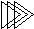Electronic Design with Excel
 Topics | VBA Basics | Back to eCircuit Center

TRIANGLE WAVE GENERATOR

SIGNAL GENERATOR

I find it fun and challenging to create waveforms using programming and the triangle wave was no exception. You can download the file here. The triangle wave can be defined by a few parameters.

V1       - Voltage level 1 (initial voltage)
V2       - Voltage level 2
T1        - Period that waveform ramps from V1 to V2
T2        - Period that waveform ramps from V2 to V1

One way to accomplish this waveform is by using voltage slopes and IF, THEN statements.

 Function Tri_Wave(t, V1, V2, T1, T2) ' ************************************************************* ' Generate Triangle Wave ' ' t - time ' V1 - voltage level 1 (initial voltage) ' V2 - voltage level 2 ' T1 - period ramping from V1 to V2 ' T2 - period ramping from V2 to V1 '*************************************************************** Dim t_tri, dV_dt1, dV_dt2 As Double Dim N As Single ' Calculate voltage rates of change (slopes) during T1 and T2 dV_dt1 = (V2 - V1) / T1 dV_dt2 = (V1 - V2) / T2 ' given t, how many full cycles have occurred N = Application.WorksheetFunction.Floor(t / (T1 + T2), 1) ' calc the time point in the current triangle wave t_tri = t - (T1 + T2) * N ' if during T1, calculate triangle value using V1 and dV_dt1 If t_tri <= T1 Then     Tri_Wave = V1 + dV_dt1 * t_tri ' if during T2, calculate triangle value using V2 and dV_dt2 Else    Tri_Wave = V2 + dV_dt2 * (t_tri - T1) End If End Function

To see the VBA code hit ALT-F11 and double click on the Modules > Module1 in the VBA Project window.

WAVEFORM PARAMETERS AND FUNCTION CALL

The description of the triangle wave is entered in these cells.

 V1 -1 V2 1 T1 0.005 T2 0.005 dT 0.0002

The time column is generated by entering the time increment dT at location C14. Each time point is simply the previous time point plus the delta, A17+\$C\$14. Note, that C14 is a fixed reference point.

The cells in the Vtri column holds the function call. The entries in this column looks like

=Tri_Wave(A17,\$C\$9,\$C\$11,\$C\$12,\$C\$10)

where A17 is the current time point and C9 through C10 hold the triangle. I still get a kick out of changing the parameters and then watching the plot change.

CREATING THE WAVEFORM

How do you create a triangle wave? The waveform simply ramps linearly from V1 to V2 during T1, and then from V2 to V1 during T2. Therefore, you need to calculate the slope for both cases.

dV_dt1 = (V2 - V1) / T1
dV_dt2 = (V1 - V2) / T2

Next, the function could create many triangle cycles. So the big question is this, where in the current cycle are you. To find out, you need to adjust the current time by subtracting off how many whole cycles (N) have already occurred.

' given t, how many full cycles have occured
N = Application.WorksheetFunction.Floor(  t/(T1 + T2), 1)

' calc the time point in the current triangle wave
t_tri = t - (T1 + T2) * N

The first calculation above calculates the ratio of current time to the triangle's period, t/(T1+T2), and then rounds down using the Floor function. (NOTE: Because VBA does not directly support the Floor function, you need to call it from Excel with the following: Application.WorksheetFunction.Floor.) The next calculation finds the time point (t_tri) in the current cycle by subtracting off N whole cycles worth of time. Now we can use t_tri to calculate the waveform.

The function uses an IF,THEN, ELSE statements to determine if t_tri occurs is period T1 or T2. If in period T1, the waveform is simply the initial voltage V1 plus t_tri multiplied by the slope dV_dt1.

If t_tri <= T1 Then
Tri_Wave = V1 + dV_dt1 * t_tri

If in period T2, the waveform is a function of V2 and the slope dV_dt2.

Else
Tri_Wave = V2 + dV_dt2 * (t_tri - T1)

Note that the time is adjusted using (t_tri - T1) to determine how much time has elapsed in T2.

Vary the parameters and see the plot change. Decrease or Increase dT to get more or less points per waveform. We'll use this ramp in later topics as inputs to circuits like amplifiers and ADCs.